Mathematics

# One to One Function

3.8k views

 1 Introduction 2 One to one Function 3 What is a one to one function 4 Number of One to one Functions 5 How to determine if a function is one to one 6 Inverse of one to one function 7 One to One Function Examples 8 Summary

12 October 2020

## Introduction

Before we discuss types of Functions and some of them in more detail. Lets quickly recap what is a Function?

We can say Functions are like mathematical objects that takes X values as Input to the machine and then gives the output as Y values or we can f(x) that is the function of X which is in one to one correspondence. We can describe this input numbers "x" as being the domain of the function, while the output numbers f(x) are the range of the function.

It is a special type of relationship where every Domain value will have only one Range Value.

There is a different type of functions, One to One function, Many One functions, Onto function, Into Function. But here we will discuss One to One function and Many to one function in detail.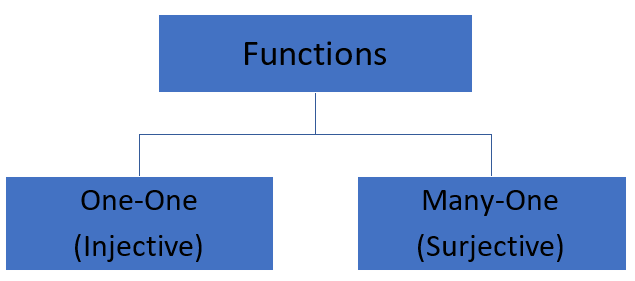The type of function depends on the mapping.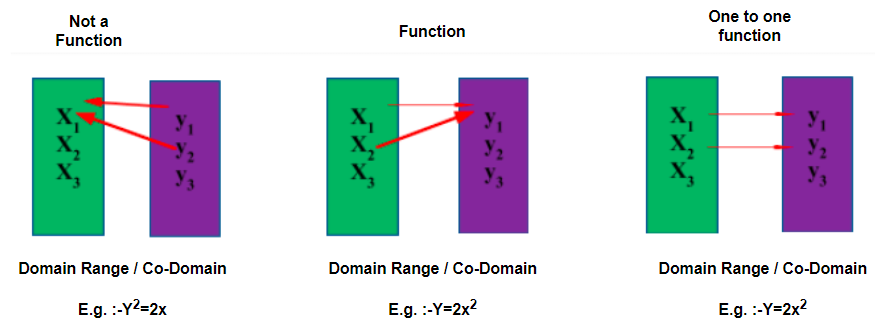## One to One Function

Referring to the above diagram C, it is a one to one function because every value of x (in Domain) when plugged into a function will get us a different single value of y (in co-domain).

## What is a one to one function?

As we have seen above as the name suggests one to one function is a function in which each of the element in the Domain will have unique mapping only with one of the elements in Codomain or also called Range/Image.

This means in a one-one function given any Y value there is only one X that can be paired with the given Y. We also call this Injective.

Example if x1≠ x2 in the domain then f(x1) ≠ f(x2) in Co- Domain too.

Let’s first understand this function with an example

Suppose there is function f(x) defined as Real to Real wherein Domain has Real numbers and Range (Co-domain/Image) also comes from Real numbers.

i.e. f(x): X (Real Numbers)  → Y (Real Numbers)

Let’s define f(x) = 3x +4

Here Domain X and Range Y are as follows: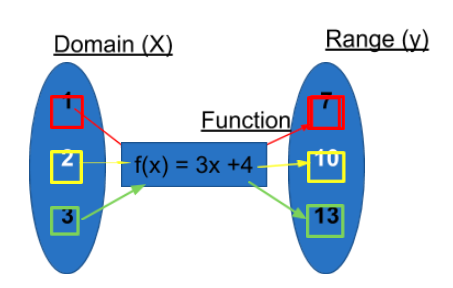In the above diagram if you see when we input 1 in the function f(x) in place of x we get the output of 7 as the Range or Image.

Similarly, when we input 2 and 3 in place of x in the above function f(x) = 3x +4, we will get the output of 10 and 13 respectively.

So here if we see for every one element of input there is only one output or image in the range section. We will never see that one element of input will have two or more outputs. For reference from the above example input of just 2 in the above function cannot have 7 and 10 both as output.

If that happens then it is not a one-one function or a function at all.

This is what is called one to one function.

### Many to One Function

When we have one or the same value as output for two or more input of real number then it is called Many to One Function.

So, let's say if we consider f(x)= x2 then if we replace x with 1, we get the output as (1)2 =1.

Similarly, when we replace x with -1 then also, we get the output as (-1) 2 =1. This would mean for two different Input values we are getting the same output value/number.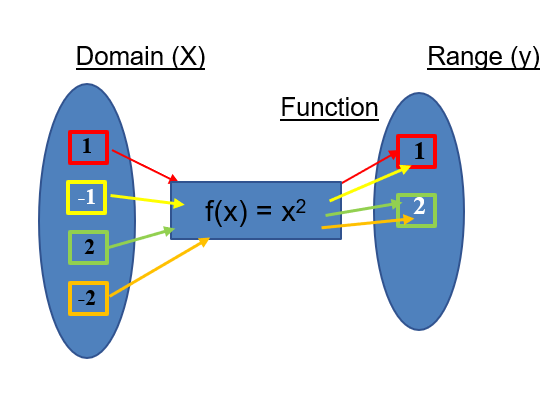Referring to the above diagram and function we see that with more than one input in the function we get only one output and is called Many to One Function i.e. many elements have only one image or value.

Example: In a classroom, many students are mapped to a single teacher. This is a common many to one function example.

## Number of One to One Functions

The number of one to one functions would mean the number of possible or ways in which each of the elements in Domain can be mapped uniquely or exclusively to elements in Co-Domain.

Let’s understand this with an

 Example 1

Suppose we have x and y in Domain. 1 and 2 in Co -Domain.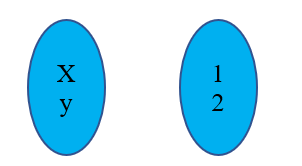How many possible one-to-one function you can think of from x, y to 1,2.

To be honest its nothing but unique permutations and combinations we can have. Say with the above example the only two possible one to one functions are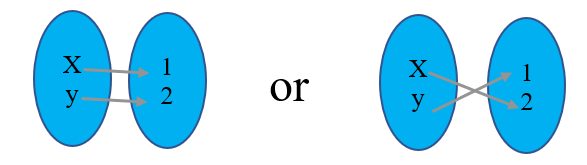So, we can say there are not infinite but finite many.

 Example 2

How many possible one-to-one function can you think of with the Given {a, b, c,} {1, 2, 3,}?

So typically, we will write this in below 6 ways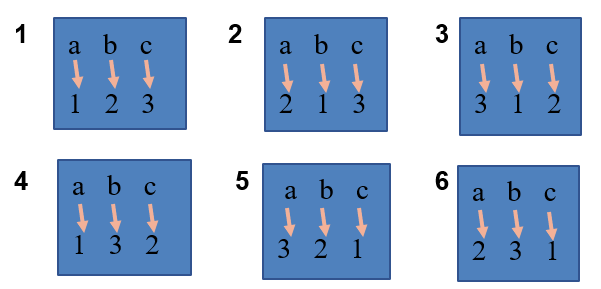So here we can say there is 3! Or 6 Of one to one functions for {a, b, c,} {1, 2, 3,}

Now if I ask you how many 6 people can get together and decide to take a photograph having 5 people at a time?

 Answer: We would write the number of one-to-one function as 6P5

## How to determine if a function is one to one

Some of the most frequently asked questions are based on how to tell if a function is one to one.

So, How to determine if a function is one to one?

There are 3 ways to check whether a function is a one-to-one function or not- Algebraic, Graphical, and Calculus.

### 1. Calculus Method

Suppose we are given a function y=f(x)

First We need to find the derivative of f(x) i.e. f'(x)

Now we have to observe that f'(x) is > 0 for the entire given interval or < 0 for the entire given interval. In both the case, we can say our given function f(x) is a one to one function.

However, if we find that within the given interval f'(x) is at time <0 or =0 and at times > 0 then it is not one to one function.

We can also see if the one-to-one function is monotonic that is strictly increasing throughout the interval or strictly decreasing throughout the interval.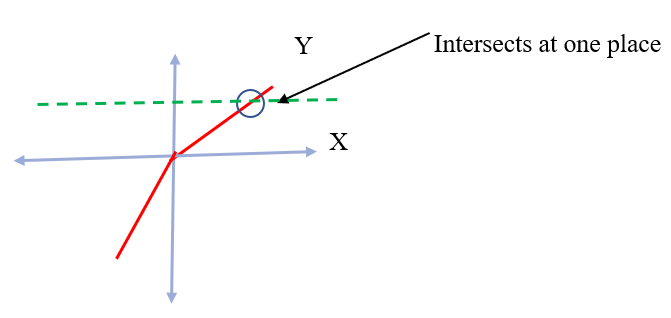Example

Here Domain is very important.

$$f: (-1,1) \rightarrow R$$

$$f(x) = x^2+2x$$

Then f’(x) =2x +2 = 2(x+1)

So, if we put x=-1/2 or 1/2 we always get value > 0

This would mean the given function is a one-to-one function.

### 2. Algebraic Method

Algebraic one to one function would mean if f(a) = f(b) which mean a=b

Now Let’s say we have a function f(x) = (3x+5)/5

Then by plugging in a and b in place of x we get

\begin{align}\frac{(3a+5)}{5} &= \frac{(3b+5)}{5}\\3a+5&= 3b+5\\3a &=3b\\a&=b \end{align}

We can say they are not 2 different values of x but the same value of x.

### 3. Graphical Method:

We already know that if a function with the input of different x value has an output of different y value then it is a one to one function.

By using the Horizontal Line Test, we can determine if the given one to one function graph represents a one-to-one function. So, if any horizontal line is going to intersect the graph of the function in exactly one point then the function is a one to one function.If some horizontal line intersects the one to one function graph more than once, then the function is not one-to-one.

## The Inverse of one to one function

The inverse function would mean the inverse of the parent function or any other function.

Let's say we have a function f(x) then the inverse function would be f-1(x).

Now let’s talk about the Inverse of one to one function.

If we have an inverse of one to one function that would mean domain of our original function f(x) = Range of Inverse f-1(x). If we have Domain of inverse of one to one function i.e. f-1(x) then that would be the Range of our original function f(x).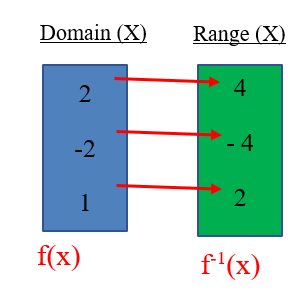There is a special property to inverse functions. If we are given two one to one functions and we think they are inverse one to one functions to one another, we can test this by plugging one function into another.

Let’s say my function is f(x) = 5x

And inverse function is f-1(x) = 1/5x then take this and plug it into original function f(x) we get

\begin{align}f(f-1(x)) &= 5\times\frac{1}{5x}\\&=x \end{align}

That would mean these are indeed inverse of each other.

### Inverse One to One Function Graph

The one to one function graph of an inverse one to one function is the reflection of the original graph over the line y = x.

The original function is = 2x + 1.

The new red line is our inverse of y = 2x + 1.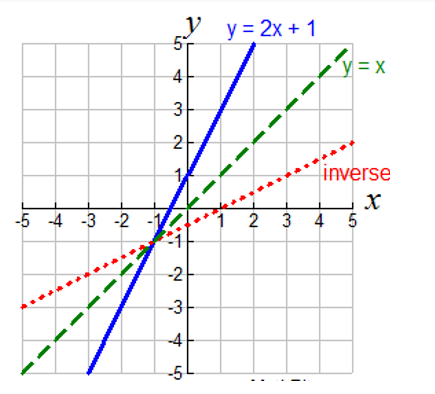Note: Not all graphs will be a function that produces inverse.

If the one to one function passes the Horizontal Line Test, its inverse will pass the Vertical Line Test for functions. In the next section let us look at some one to one function examples.

## One to One Function Examples

 Example 1

Show that f(y)=1/y is a one to one function.

Assume that there exists some y1 and y2, such that f(y1) = f(y2). i.e. 1/ 1/y1= 1/ y2

But, $$\frac{1}{y_1}= \frac{1}{y_2}$$

\begin{align}&⇒\frac{1}{y_1} - \frac{1}{y_2}=0\\&⇒ \frac{(y_1 - y_2)}{ y_1 y_2} = 0\\&⇒ \text{the numerator must equal zero! i.e. }y_2− y_1=0\\&⇒ y_2 = y_1 \end{align}

Thus it is a one-to-one function.

 Example 2

Which of the following functions are one to one functions?

(a) f (x) = 3x-2                            (b) f (x) = x2+3

Solution:

If above is the one to one function then it must satisfy the condition

f(x1) = f(x2)

a. Here in 1s equation by replacing x we get,

\begin{align}3 x_1-2 &= 3 x_2 - 2\\ 3 x_1 &= 3 x_2 \qquad \text{ Or}\\x_1 &= x_2 \end{align}

Therefore f(x) is one to one function

b. Here in 2nd equation by replacing x we get,

\begin{align}X_1^2+3 &= X_2^2+3\\ X_1^2& = X_2^2\\X_1 &≠ X_2 \end{align}

Therefore f(x) is not one to one function.

## Summary

Functions are very important as they can be applied in algebra, calculus, science, and engineering. It can also be applied to our day to day life.

If we look around, we can find many such examples of one to one relationship and real-life examples one to one function examples:

• One family stay in one house, and the house has one family.
• One person has one passport, and that passport belongs to one person.
• One person has one name, and that name belongs to one person.
• Each student gets one desk which can only be used by one student.
• A company creates one product, and that product is only made by that company.
• A CEO hires only one personal assistant, and that assistant only works with that CEO.
• People have unique fingerprints, which only belong to that individual.

And thus, it makes it important for us to study one to one functions, How to determine if a function is one to one, and various properties related to it.

Written by Rashi Murarka

GIVE YOUR CHILD THE CUEMATH EDGE
Access Personalised Math learning through interactive worksheets, gamified concepts and grade-wise courses